Next: Faraday Rotation Up: Wave Propagation in Uniform Previous: High Frequency Limit

# Polarization of Electromagnetic Waves

The electric component of an electromagnetic plane wave can oscillate in any direction normal to the direction of wave propagation (which is parallel to the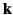-vector). Suppose that the wave is propagating in the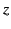-direction. It follows that the electric field can oscillate in any direction that lies in the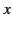-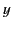plane. The actual direction of oscillation determines the polarization of the wave. For instance, a vacuum electromagnetic wave of angular frequency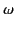that is polarized in the-direction has the associated electric field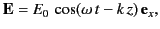(813)

where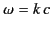. Likewise, a wave polarized in the-direction has the electric field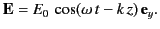(814)

These two waves are termed linearly polarized, because the electric field vector oscillates in a straight-line. However, other types of polarization are possible. For instance, if we combine two linearly polarized waves of equal amplitude, one polarized in the-direction, and one in the-direction, that oscillate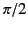radians out of phase, then we obtain a circularly polarized wave: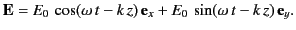(815)

This nomenclature arises from the fact that the tip of the electric field vector traces out a circle in the plane normal to the direction of wave propagation. To be more exact, the previous wave is a right-hand circularly polarized wave, because if the thumb of the right hand points in the direction of wave propagation then the electric field vector rotates in the same sense as the fingers of this hand. Conversely, a left-hand circularly polarized wave takes the form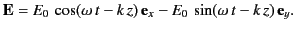(816)

Finally, if the- and-components of the electric field in the previous two expressions have different (non-zero) amplitudes then we obtain right-hand and left-hand elliptically polarized waves, respectively. This nomenclature arises from the fact that the tip of the electric field vector traces out an ellipse in the plane normal to the direction of wave propagation.Next: Faraday Rotation Up: Wave Propagation in Uniform Previous: High Frequency Limit
Richard Fitzpatrick 2014-06-27